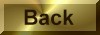Practice Problem 7

Determine the molecular weight of acetic acid if a solution that contains 30.0 grams of acetic acid per kilogram of water freezes at -0.93oC. Do these results agree with the assumption that acetic acid has the formula CH3CO2H?

Solution

The freezing point depression for this solution is equal to the difference between the freezing point of the solution (-0.93oC) and the freezing point of pure water (0oC).TFP = -0.93oC - 0.0oC = -0.93oC

We now turn to the equation that defines the relationship between the freezing point depression and the molality of the solution.TFP = - kfm

Since we know the change in the freezing point, and we can look up the freezing point depression constant in a table, we have enough information to calculate the molality of the solution.

 m =TFP = 0.93oC = 0.50 m kf 1.853oC/m

At this point, we might return to the statement of the problem, to see if we are making any progress toward an answer. According to this calculation, there are 0.50 moles of acetic acid per kilogram of water in this solution. The problem stated that there were 30.0 grams of acetic acid per kilogram of water in the solution. Since we simultaneously know the number of grams and the number of moles of acetic acid in this sample, we can calculate the molecular weight of acetic acid.

 30.0 g = 60 g/mol 0.50 mol

The results of this experiment are in good agreement with the molecular weight (60.05 g/mol) expected if the formula for acetic acid is CH3CO2H.